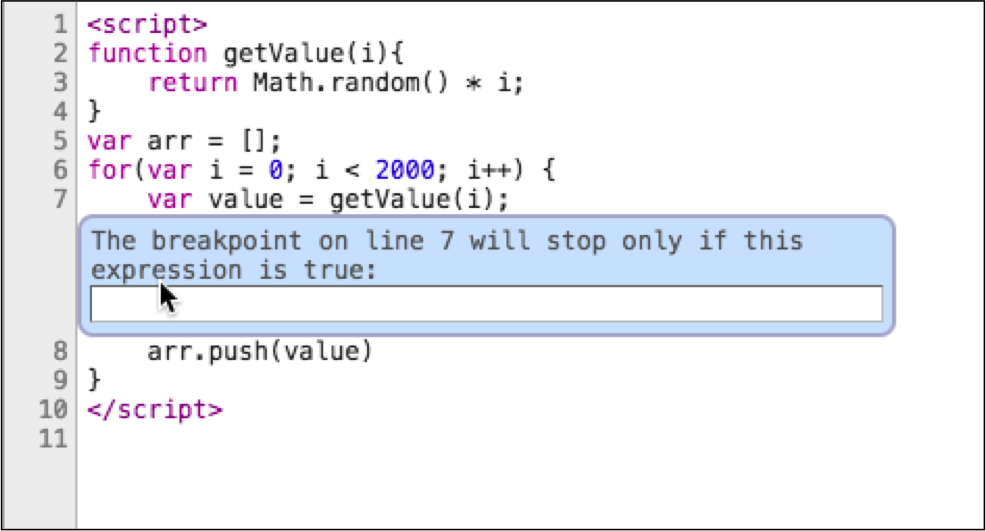# Conditional `page`

Learn how to set a conditional breakpoint.

## The problem

``````<script src="//bitovi.github.io/academy/static/scripts/debugging/variables.js"></script>
<script type="module">
function conditional(){
let sum = 0;
for(let i = 0; i < 2000; i++) {
var conditionalValue = getConditionalValue(i);
sum += conditionalValue;
}
console.log("What does getConditionalValue() return when `i` is 1000?");
debugger;
return sum;
}
conditional();
</script>
``````

## What you need to know

Often you only want to break when your app is at a certain state. The following code creates 2000 random numbers:

``````function getValue(i){
return Math.random() * i;
}
var arr = [];
for(var i = 0; i < 2000; i++) {
var value = getValue(i);
arr.push(value)
}
``````

Chrome lets you add conditional breakpoints that break when an expression evaluates to truthy. Right click the line number you'd like to break on and enter the expression.## The solution

The answer is `u`.